# Hay’s bridge for measurement of inductance

## Hay’s bridge for measurement of inductance :

The Hay’s bridge is modification of the Maxwell’s bridge. The connection diagram of the Hay’s bridge is shown in figure below. This Hay's bridge uses a resistor in series with a standard capacitor (unlike the Maxwell’s bridge which uses a resistance in parallel with the capacitor).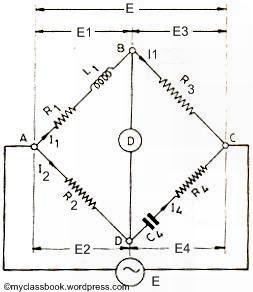Hay's bridge for measurement of inductance

Let

L1=unknown resistance having a resistance R1,

R2, R3, R4=known non-inductive resistance,

C4=standard capacitor.

At balance,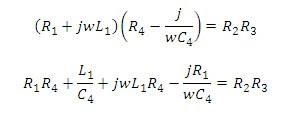Separating real and imaginary term, we obtain: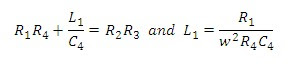Solving the above two equations we have,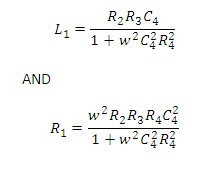The Q factor of the coil is :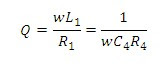### Advantages of the Hay’s bridge:

1)      This bridge gives very simple expression for unknown inductance for high Q coils, and is suitable for coils having Q > 10.

2)      This bridge also gives the simple expression for Q factor.

3)      From expression of Q factor it is clear that for high Q factor the value of resistance R4 should be small.

1)      The Hay’s bridge is suited for measurement of high Q inductors, specially those inductors having Q > 10. For inductors having Q values smaller than 10, the term (1/Q)^2 in the expression for inductance L1 becomes rather important and thus cannot be neglected. Hence this bridge is not suited for measurement of coils having Q less than 10 and for thse applications a Maxwell's bridge is more suited.

1.2.3.